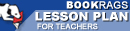Lesson Plans

# Chaos: Making a New Science Quiz | Eight Week Quiz F

This set of Lesson Plans consists of approximately 131 pages of tests, essay questions, lessons, and other teaching materials.
 View a FREE sampleName: _________________________ Period: ___________________

This quiz consists of 5 multiple choice and 5 short answer questions through chapters 7-8.

## Multiple Choice Questions

1. In statistics, what refers to any statistical relationship between two random variables or two sets of data?
(a) Commonality.
(b) Diversity.
(c) Prediction.
(d) Dependence.

2. What mathematical term refers to a bifurcation in which the system switches to a new behavior with twice the period of the original system in a discrete dynamical system?
(a) Dynamic equilibrium.
(b) The Navier-Stokes equation.
(c) Period doubling bifurcation.
(d) Software visualization.

3. When was Steven Smale born?
(a) 1930.
(b) 1943.
(c) 1938.
(d) 1932.

4. What can be formed by taking a finite Cartesian product of the Cantor set with itself, making it a Cantor space?
(a) Cantor dust.
(b) Fractal.
(c) Helium in a Box.
(d) Euclidean space.

5. What is defined as a mess of disorder on all scales and is not stable?
(a) Energy.
(b) Turbulence.
(c) Viscosity.
(d) Fractal.

1. Who is attributed with the following quote from Chapter 8, "Images of Chaos": "What else, when chaos draws all forced inward to shape a single leaf"?

2. What is a concept in mathematics where a fixed rule describes the time dependence of a point in a geometrical space?

3. In Chapter 1, "The Butterfly Effect," the author writes, "The Butterfly Effect acquired a technical name: sensitive dependence on _____________"?

4. What were the subjects allowed to glance at one at a time in the experiment from the 1940s described by Gleick in Chapter 2, "Revolution"?

5. In control theory, a system is _____ if any of the roots of its characteristic equation has real part greater than zero.

 This section contains 258 words (approx. 1 page at 300 words per page) View a FREE sample